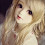## Van Parcel Scooter Puzzle

This is a very tricky math puzzle, but try to solve it without others help. Mathematical puzzles are a great way to challenge and exercise your brain. Solving these type of puzzles can help to improve your overall concentration and focus, which can be beneficial in other areas of your life.

HINT: Look at each objects for any multiple images.

Scroll down to see the correct answer with explanation.

Explanation
Equation 1: 14 + 14 + 14 = 42  (Here we get the value of VAN = 14)

Equation 2: 14 + 8 + 8 = 30  (Here we get the value of a single PARCEL = 2. But since there are 4 set of Parcels, its value becomes 8)

Equation 3: 6 + 8 + 4 = 18   (Here we get the value of a SCOOTER = 4. But if you look closely you can see there are two scooters (one behind another) in the middle part of the equation)

Equation 4: 14 + 4 + 8 = 26

1.2.